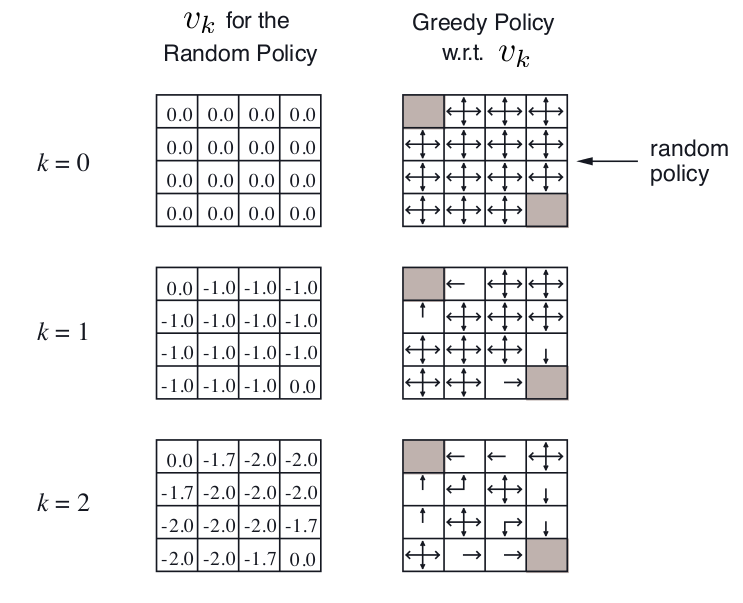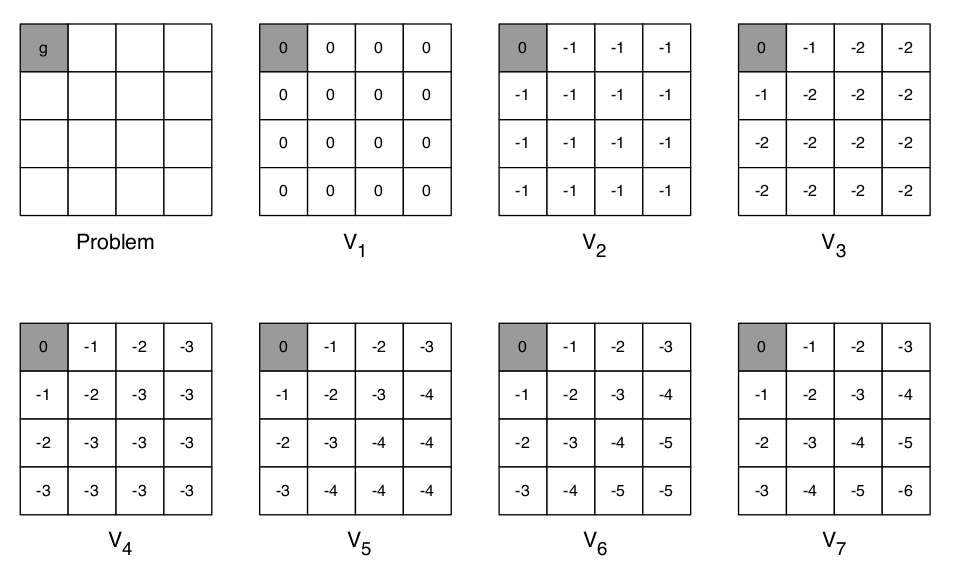【转载请注明出处】chenrudan.github.io#### 1.内容回顾

Bellman方程 形式
Bellman Expectation Equation $v_{\pi}(s) = \sum_{a\in A}\pi(a\s)q_{\pi}(s,a) =\sum_{a\in A}\pi(a\s)(R_s^a + \gamma \sum_{s’\in S}P_{ss’}^av_{\pi}(s’))$
Bellman Expectation Equation $q_{\pi}(s,a) = R_s^{a} + \gamma \sum_{s’ \in S}P_{ss’}^av_\pi(s’)=R_s^a + \gamma \sum_{s’\in S}P_{ss’}^a\sum_{a’\in A}\pi(a’\s’)q_{\pi}(s’,a’)$
Bellman Optimality Equation $v_*(s) = \underset{a}{max}q_*(s,a) = \underset{a}{max} R_s^{a} + \gamma \sum_{s’ \in S}P_{ss’}^av_*(s’)$
Bellman Optimality Equation $q^*(s,a) = R_s^{a} + \gamma \sum_{s’ \in S}P_{ss’}^av_*(s’) = R_s^a + \gamma \sum_{s’\in S}P_{ss’}^a \underset{a’} {max}q^*(s’, a’)$

#### 2. 动态规划与Planning

MDP需要解决的问题有两种，第一种是prediction，它已知MDP的$S,A,P,R,\gamma$以及policy，目标是算出在每个状态下的value function，即处于每个状态下能够获得的reward是多少。而第二种是control，它已知MDP的$S,A,P,R,\gamma$但是policy未知，因此它的目标不仅是计算出最优的value function而且要给出最优的Policy。

#### 3. Policy Iteration

• Policy Evaluation:基于当前的Policy计算出每个状态的value function
• Policy Improvment:基于当前的value function，采用贪心算法来找到当前最优的PolicyPolicy Iteration会一直迭代到收敛，具体证明过程可以去看视频(46:09起)。#### 3. Value IterationPrediction Bellman Expectation Equation Iterative Policy Evaluation
Control Bellman Expectation Equation + Greedy Policy Improvement Policy Iteration
control Bellman Optimality Equation Value Iteration